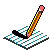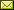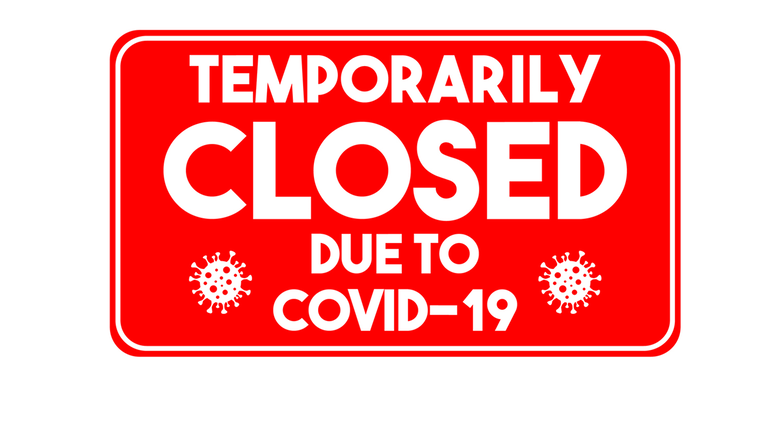PHYSICS@HORTONPhysics12

Physics 11

Math 11

NS Exams

Curriculum

Tips

Tutorials

McGraw Hill

Resources

Need it?

News

Enrichment

GiancoliIB Physics SL

April 2021 Notes

 April 1 Projectile Practice from McGraw-Hill (see post in Google Classroom) Notes April 6 Error Analysis Lab Motion on an Incline (not in 2021) Investigating the Fall of a Coffee Filter (Design) (not in 2021) April 7 PRESCRIBED PRACTICAL ~ Measuring the acceleration due to gravity by timing a freefalling object (write up as DCP/CE) Try the following simulation: Free Fall Model April 8 QUIZ (2.1 Kinematics) work on Labs April 9 Forces (Weight/Normal Force/FBDs) BrainPopUK: Gravity What is Gravity? What is a Force? What Forces are Acting on You? Forces and Motion Simulation Lab Notes April 10 April 12 Forces (Resultant Forces/Balanced & Unbalanced Forces/Newton's First Law/Translational Equilibrium/Newton's Second Law) Notes April 13 Forces & Motion: Basics (Tug of War & Acceleration Lab) (needs Java) Finish "Popper Physics" Finish "Error Analysis" and "Freefall" Work on "TO DO" list April 14 Forces (Newton's Second Law) Brain PopUK: Force Negative Mass?? Notes April 15 Forces (Friction) Forces (Fluid Resistance) Forces in 1D (needs Java) The Physics Classroom The Calculator Pad Notes April 16 Investigating the Terminal Velocity of a Coffee Filter April 19 Work on Terminal Velocity Lab April 20 Momentum & Impulse (Momentum, Impulse & Newton's Second Law/F-t graphs/Conservation of Linear Momentum) Momentum & Impulse More Momentum & Impulse Impulse Bouncing Ball Momentum & Collisions (PHYSCLIPS) What is wrong in the NS Driver's Handbook? (see p51) Notes April 21 Momentum & Impulse (Conservation of Linear Momentum) pHET Collision Lab (settings: 1D, check velocity vectors & momenta diagram, try 100%, 50%, 0% elasticity, try different mass combinations, try different velocities and look at the total mometum before & after collision) 2D Collisions (World-in-Motion video analysis) April 22 Momentum & Impulse (Conservation of Linear Momentum) Cannon Ball Fired from Back of Moving Truck Recoil Velocity Animation Notes1 Notes2 Momentum & Impulse (Conservation of Linear Momentum) Notes Video: Momentum (Vector Addition) ~ see Google Classroom April 23 Finish up lab work: Terminal Velocity Lab pHET Collision Lab 2D Collisions (World-in-Motion video analysis) April 26 Momentum & Impulse (Newton's Third Law) Notes April 27 Work, power, and energy (Forms of energy, conservation of energy, work) BrainPop: Work BrainPop: Inclined Plane Notes April 28NO SCHOOL April 29NO SCHOOL (originally scheduled as Inservice/Parent-Teacher Day) April 30Learning from Home Work, power, and energy (Work, force displacement graphs, Hooke's Law) Hooke's Law Animation Hooke's Law Spring Virtual Hooke's Law Lab Hooke's Law (showing elastic limit) PhET: Hooke's Law Find Ronald's Mass Notes

{subdirectory}